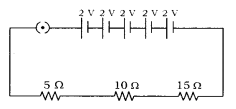# (i) Draw X schematic diagram of a circuit consisting of a battery of five 2V cells

(i) Draw X schematic diagram of a circuit consisting of a battery of five 2V cells, a 5 ohm resistor, a 10 ohm resistor and a 15 ohm resitor, and a plug key, all connected in series.
(ii) Calculate the electric current passing through the above circuit when the key is closed.

(i)(ii) When the key is closed, total voltage applied across the circuit will be = 2 × 5V = 10V Total resistance (R ) applied across the circuit in Ohms = 5 + 10 + 15 = 30Ω